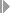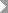Show TOC

###(10) Inventory Determination, Without Planned Costs, Without Milestone Billing#### Use

You can use the method Inventory Determination, Without Planned Costs, Without Milestone Billing for:

• Sales orders

• Projects

You can use the method Inventory Determination, Without Planned Costs, Without Milestone Billing to:

• Calculate inventories in the amount of the actual costs if the actual revenue is zero

• Calculate the cost of sales in the amount of actual costs if actual revenue is not zero

The inventory is canceled as soon as actual revenues are received.

Note the following:

• You cannot create reserves.

• This method is unsuitable for milestone billing because such billings result in final cancellation of the capitalized costs.

• There is no proportionality between the cost of sales and the revenue:

As soon as revenue is received, the actual costs are considered cost of sales in full. In other words, the cost of sales is not proportional to actual revenues as with the revenue-based method, nor is it proportional to the quantity sold as with the quantity-based method.

• You do not need any planned data.

• Sales and administration costs are always reported and settled as cost of sales.

#### Prerequisites

Choose a results analysis method in simplified Customizing for Product Cost by Sales Order underPeriod-End ClosingResults AnalysisValuation Method.

#### Features

If R(a) = 0 then C(PA) = 0 and C(z) = C(a)

If R(a) <> 0 then C(PA) = C(a) and C(z) = 0

R(PA) = R(a)

#### Example

Period 01

In period 01 you have actual costs of USD 20,000 but no revenues. In results analysis, the system calculates the following data:

• Cost of sales affecting net income of USD 0

• Revenue affecting net income of USD 0

• Capitalized costs of USD 20,000

You then settle the following:

• The capitalized costs to FI and EC-PCA

• No cost of sales and no revenues to CO-PA

The following values are reported in CO-PA:

Profitability Analysis

 Actual revenues 0 Calculated cost of sales 0 Profit 0

The income statement shows the following values:

Income Statement

 Expense Revenue Actual costs 20,000 Inventory increase Capitalized costs 20,000 20,000 20,000

Period 02

In period 02 actual costs increase to USD 130,000. You deliver to your customer and send an invoice for USD 200,000. The order is now fully delivered and fully invoiced. In results analysis, the system calculates the following data:

• Cost of sales affecting net income of USD 130,000

• Revenue affecting net income of USD 200,000

• Capitalized costs of USD 0

You then settle the following:

• The cost of sales to CO-PA

• The revenues to CO-PA

• The cancellation of the capitalized costs to FI and EC-PCA

The following values are reported in CO-PA:

Profitability Analysis

 Revenues (actual revenues) 200,000 Cost of sales (actual costs) 130,000 Profit 70,000

The income statement shows the following values:

Income Statement

 Expense Revenue Actual costs 130,000 Actual revenues 200,000 Profit 70,000 200,000 200,000

The profit on your sales order is USD 70,000.